# Mathematics Program

### Algebra Group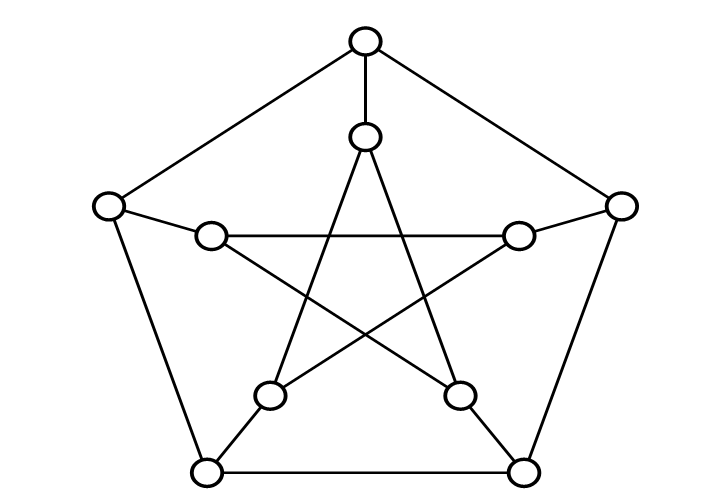Number Theory, Algebraic Geometry, Arithmetic Geometry, Motives, Singularities, Group Theory, Representation Theory, Commutative Algebra, Arithmetic Fundamental Group, Random Number Generation, Quasi-Monte Carlo Method, Coding Theory, Cryptography

### Geometry and Topology Group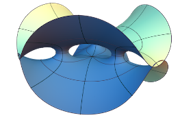Differential Geometry, Topology, Manifolds, 3 and 4 Dimensional Mathematics, Knots, Hyperbolic Geometry, Homogeneous Spaces, Symmetric Spaces, Representation Theory of Lie Groups, Singularities

### Mathematical Analysis Group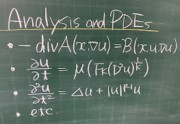Differential Equations, Nonlinear Analysis, Dynamical Systems, Potential Theory, Complex Analysis, Scattering Theory, Algebraic Analysis, Asymptotic Analysis, Resurgence Theory

### Probability Theory and Mathematical Statistics Group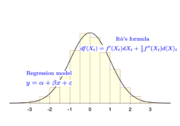Probability Theory, Stochastic Processes, Financial and Insurance Mathematics, Random Fields, Theory for Multivariate Data Analysis and its Applications, Statistical Inference, Asymptotic Expansion for Statistical Distributions, Resampling Methods, Mathematical Statistics

### Mathematical Sciences Group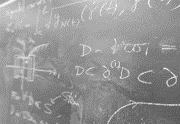Differential Geometry, Differential Equations,Bayesian Statistics, Knot Theory

up